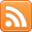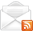# ASP.NET,C#.NET,VB.NET,JQuery,JavaScript,Gridview

aspdotnet-suresh offers C#.net articles and tutorials,csharp dot net,asp.net articles and tutorials,VB.NET Articles,Gridview articles,code examples of asp.net 2.0 /3.5,AJAX,SQL Server Articles,examples of .net technologies

### Asp.Net Encrypt and Decrypt QueryString Parameter Values in C#, VB.NET

Jun 21, 2016
Introduction

Here I will explain how to encrypt and decrypt querystring values in
asp.net using c#, vb.net with example or asp.net encrypt decrypt querystring values using c#, vb.net with example or encrypting and decrypting querystring values in asp.net using c#, vb.net with example. By using “Cryptography” properties we can encrypt and decrpy querystring values easily in asp.net website using c#, vb.net.

Description:

In previous articles I explained encrypt and decrypt password in sql server, asp.net encrypt and decrypt connection string in web.config file,
bind gridview using jquery json method in asp.net, show multiple markers in google map from database in asp.net and many articles relating to gridview, asp.net, c#,vb.net and jQuery. Now I will explain how to get encrypt and decryp querstring values in asp.net using c#, vb.net with example.

To encrypt and decrypt query string values in asp.net we are going to use two pages one is EncryptQueryString.aspx and DecryptQueryString.aspx now open your EncryptQueryString.aspx page and write the following code

EncryptQueryString.aspx

 Encrypt and Decrypt QueryString Parameter Values in ASP.NET
After completion of aspx page add following namespaces in codebehind file (EncryptQueryString.aspx.cs)

C# Code

 using System; using System.Web; using System.Text; using System.IO; using System.Security.Cryptography;
After adding namespaces write the code like as shown below

 protected void btnredirect_Click(object sender, EventArgs e) { string userid = HttpUtility.UrlEncode(Encrypt(txtId.Text.Trim())); string username = HttpUtility.UrlEncode(Encrypt(txtName.Text.Trim())); Response.Redirect("~/DecryptQueryString.aspx?userid="+userid+"&uname="+username+""); } public static string Encrypt(string inputText) { string encryptionkey = "SAUW193BX628TD57"; byte[] keybytes = Encoding.ASCII.GetBytes(encryptionkey.Length.ToString()); RijndaelManaged rijndaelCipher = new RijndaelManaged(); byte[] plainText = Encoding.Unicode.GetBytes(inputText); PasswordDeriveBytes pwdbytes = new PasswordDeriveBytes(encryptionkey, keybytes); using (ICryptoTransform encryptrans = rijndaelCipher.CreateEncryptor(pwdbytes.GetBytes(32), pwdbytes.GetBytes(16))) { using (MemoryStream mstrm = new MemoryStream()) { using (CryptoStream cryptstm = new CryptoStream(mstrm, encryptrans, CryptoStreamMode.Write)) { cryptstm.Write(plainText, 0, plainText.Length); cryptstm.Close(); return Convert.ToBase64String(mstrm.ToArray()); } } } }
VB.NET Code

 Imports System.Web Imports System.Text Imports System.IO Imports System.Security.Cryptography Partial Class VBCode Inherits System.Web.UI.Page Protected Sub Page_Load(ByVal sender As Object, ByVal e As EventArgs) End Sub Protected Sub btnredirect_Click(ByVal sender As Object, ByVal e As EventArgs) Dim userid As String = HttpUtility.UrlEncode(Encrypt(txtId.Text.Trim())) Dim username As String = HttpUtility.UrlEncode(Encrypt(txtName.Text.Trim())) Response.Redirect((Convert.ToString((Convert.ToString("~/DecryptQueryString.aspx?userid=") & userid) + "&uname=") & username) + "") End Sub Public Shared Function Encrypt(ByVal inputText As String) As String Dim encryptionkey As String = "SAUW193BX628TD57" Dim keybytes As Byte() = Encoding.ASCII.GetBytes(encryptionkey.Length.ToString()) Dim rijndaelCipher As New RijndaelManaged() Dim plainText As Byte() = Encoding.Unicode.GetBytes(inputText) Dim pwdbytes As New PasswordDeriveBytes(encryptionkey, keybytes) Using encryptrans As ICryptoTransform = rijndaelCipher.CreateEncryptor(pwdbytes.GetBytes(32), pwdbytes.GetBytes(16)) Using mstrm As New MemoryStream() Using cryptstm As New CryptoStream(mstrm, encryptrans, CryptoStreamMode.Write) cryptstm.Write(plainText, 0, plainText.Length) cryptstm.Close() Return Convert.ToBase64String(mstrm.ToArray()) End Using End Using End Using End Function End Class
Now open DecryptQueryString.aspx and write the code like as shown below

DecryptQueryString.aspx

 Decrypt QueryString in Asp.Net
Decrypted Values:

User Name : User Id:
After completion of aspx page add following namespaces in codebehind (DecryptQueryString.aspx.cs)

C# Code

 using System; using System.Web; using System.Text; using System.Security.Cryptography; using System.IO;
After adding namespaces write the code like as shown below

 protected void Page_Load(object sender, EventArgs e) { string userid = HttpUtility.UrlDecode(Request.QueryString["userid"]); string username = HttpUtility.UrlDecode(Request.QueryString["uname"]); lblId.Text = Decrypt(userid); lblName.Text = Decrypt(username); } public static string Decrypt(string encryptText) { string encryptionkey = "SAUW193BX628TD57"; byte[] keybytes = Encoding.ASCII.GetBytes(encryptionkey.Length.ToString()); RijndaelManaged rijndaelCipher = new RijndaelManaged(); byte[] encryptedData = Convert.FromBase64String(encryptText.Replace(" ", "+")); PasswordDeriveBytes pwdbytes = new PasswordDeriveBytes(encryptionkey, keybytes); using (ICryptoTransform decryptrans = rijndaelCipher.CreateDecryptor(pwdbytes.GetBytes(32), pwdbytes.GetBytes(16))) { using (MemoryStream mstrm = new MemoryStream(encryptedData)) { using (CryptoStream cryptstm = new CryptoStream(mstrm, decryptrans, CryptoStreamMode.Read)) { byte[] plainText = new byte[encryptedData.Length]; int decryptedCount = cryptstm.Read(plainText, 0, plainText.Length); return Encoding.Unicode.GetString(plainText, 0, decryptedCount); } } } }
VB.NET Code

 Imports System.Web Imports System.Text Imports System.IO Imports System.Security.Cryptography Partial Class VBCode Inherits System.Web.UI.Page Protected Sub Page_Load(ByVal sender As Object, ByVal e As EventArgs) Handles Me.Load Dim userid As String = HttpUtility.UrlDecode(Request.QueryString("userid")) Dim username As String = HttpUtility.UrlDecode(Request.QueryString("uname")) lblId.Text = Decrypt(userid) lblName.Text = Decrypt(username) End Sub Public Shared Function Decrypt(ByVal encryptText As String) As String Dim encryptionkey As String = "SAUW193BX628TD57" Dim keybytes As Byte() = Encoding.ASCII.GetBytes(encryptionkey.Length.ToString()) Dim rijndaelCipher As New RijndaelManaged() Dim encryptedData As Byte() = Convert.FromBase64String(encryptText.Replace(" ", "+")) Dim pwdbytes As New PasswordDeriveBytes(encryptionkey, keybytes) Using decryptrans As ICryptoTransform = rijndaelCipher.CreateDecryptor(pwdbytes.GetBytes(32), pwdbytes.GetBytes(16)) Using mstrm As New MemoryStream(encryptedData) Using cryptstm As New CryptoStream(mstrm, decryptrans, CryptoStreamMode.Read) Dim plainText As Byte() = New Byte(encryptedData.Length - 1) {} Dim decryptedCount As Integer = cryptstm.Read(plainText, 0, plainText.Length) Return Encoding.Unicode.GetString(plainText, 0, decryptedCount) End Using End Using End Using End Function End Class
Demo

 If you enjoyed this post, please support the blog below. It's FREE! Get the latest Asp.net, C#.net, VB.NET, jQuery, Plugins & Code Snippets for FREE by subscribing to our Facebook, Twitter, RSS feed, or by email.Subscribe by RSSSubscribe by Email

Maitreya said...

Just love this! Keep going man!!!Anonymous said...

can u explain which algorithm is used in the encrypt and decrypt